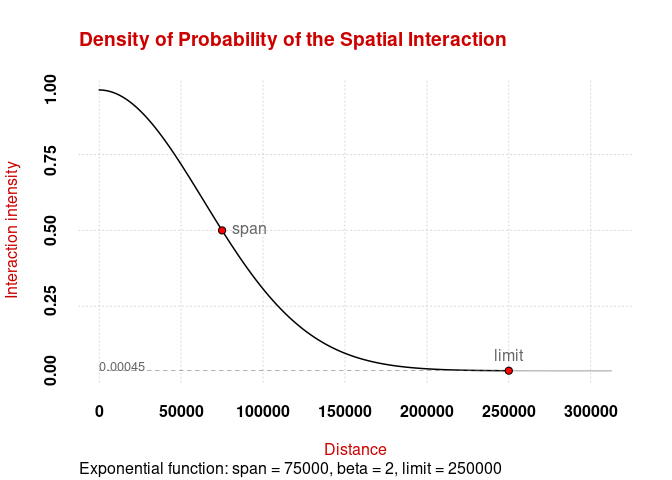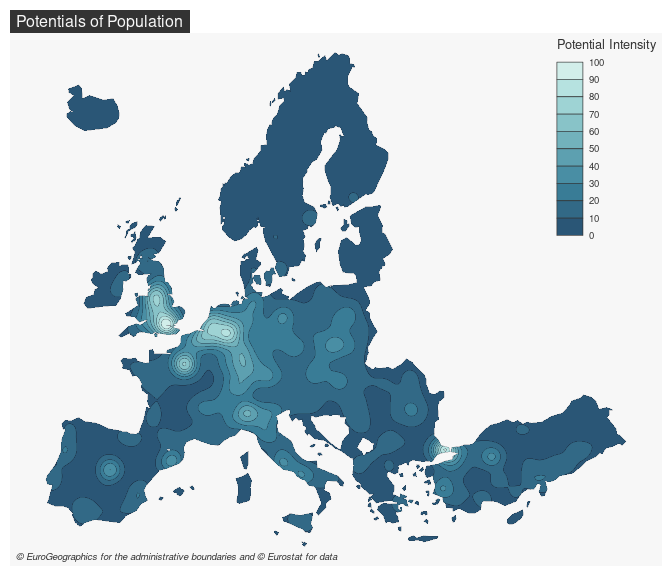This package provides functions to compute the potential model as defined by Stewart (1941). Several options are available to customize the model, for example it is possible to fine-tune the distance friction functions or to use custom distance matrices. Some computations are parallelized to improve their efficiency.

## Installation

You can install the released version of potential from CRAN with:

install.packages("potential")

You can install the development version of potential from GitHub with:

# install.packages("remotes")
remotes::install_github("riatelab/potential")

## Demo

library(sf)
library(potential)
library(cartography)
# Display the spatial interaction function
plot_inter(fun = "e", span = 75000, beta = 2, limit = 250000)# create a regular grid
y <- create_grid(x = n3_poly, res = 20000)
# compute potentials
pot <- mcpotential(
x = n3_pt, y = y,
var = "POP19",
fun = "e", span = 75000,
beta = 2, limit = 250000,
ncl = 2
)
# Define potential according to the maximum value
y\$pot <- pot / max(pot) * 100
# create equipotential areas
equipot <- equipotential(y, var = "pot", mask = n3_poly)
# map potentials
opar <- par(mar = c(0, 0, 1.2, 0), bg = "#b5bece", no.readonly = TRUE)
choroLayer(equipot, var = "center",
breaks = seq(0,100,length.out = 11),
col = hcl.colors(10, 'teal'),
border = "#121725",
lwd = .2,
legend.pos = "bottom",
legend.title.txt = "Potential Intensity",
legend.horiz = TRUE)
layoutLayer(title = "Potentials of Population",
col = "#121725", coltitle = "#4dB8da",
par(opar)This package provides access to the revamped potential-related functions initially offered by SpatialPosition.